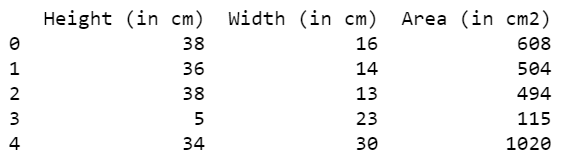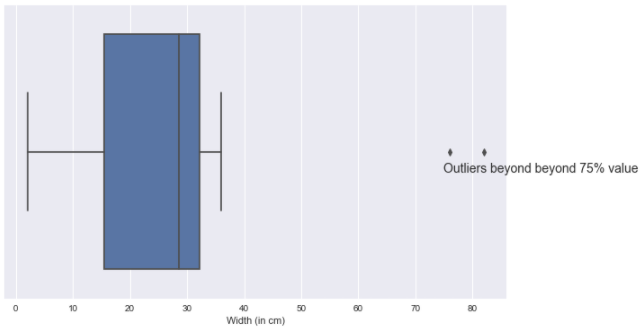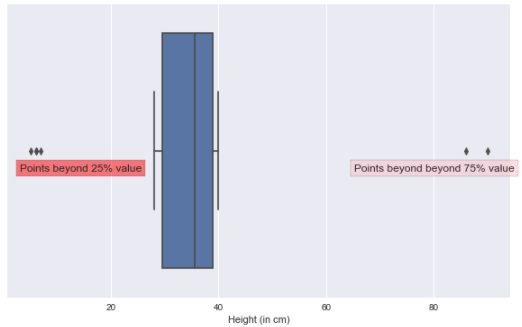# How to display text on boxplot in Python?

• Last Updated : 21 Jul, 2021

Boxplot is an important graphical plot that can be used to get a summary of data present in numerical form. The plot can give us information about statistical measures such as percentile, median, minimum and maximum values of the numerical data. In the box plot, the line which passes through the center of the box represents the median value. In this article, we will be learning about how to add plot a boxplot and display text on the boxplot.

Firstly, to create boxplot we’ll use the seaborn library, and to display text on the boxplot we will be using the text() method available inside the matplotlib.pyplot class. The text() method enables us to write strings on the plots and add customizations to them.

Let’s plot a simple boxplot at first and add some text over it.

## Python

 `# import modules` `import` `numpy as np` `import` `pandas as pd` `import` `seaborn as sns` `import` `matplotlib.pyplot as plt` `plt.style.use(``'seaborn'``)`   `# Reading the dataset` `data ``=` `pd.read_csv(``'Dataset.csv'``)` `print``(``"The shape of the dataframe is: "``,data.shape)`

Output:

`The shape of the dataframe is:  (20, 3)`

Printing the first five rows in the dataset:

## Python

 `# display data` `print``(data.head())`

Output:## Python

 `# depict box plot visualization` `plt.figure(figsize``=``(``10``, ``6``))` `sns.boxplot(data[``'Width (in cm)'``])` `plt.text(``75``, ``0.07``, ``'Outliers beyond beyond 75% value'``, fontsize``=``14``)` `plt.show()`

Output:We can observe from the above-written code, that plt.text() method was used to display the desired text that we want. It requires three compulsory positional arguments:

Syntax: plt.text(x, y, text)

Parameters:

• x-coordinate: denotes the location of the text on x-axis
• y-coordinate: denotes the location of text on y-axis
• text: denotes the string that we want to insert.

To make our box plot look more appealing we can pass many other optional parameters for formatting the text which has been discussed in the next plot.

## Python

 `# add text` `plt.figure(figsize``=``(``10``, ``6``))` `sns.boxplot(data[``'Height (in cm)'``])`   `plt.text(``3``, ``0.07``, ` `         ``'Points beyond 25% value'``, ` `         ``bbox``=``dict``(facecolor``=``'red'``, ` `                   ``alpha``=``0.5``), ` `         ``fontsize``=``12``)`   `plt.text(``95``, ``0.07``, ` `         ``'Points beyond beyond 75% value'``, ` `         ``bbox``=``dict``(facecolor``=``'pink'``, ` `                   ``alpha``=``0.5``),` `         ``horizontalalignment``=``'right'``, ` `         ``fontsize``=``12``)`   `plt.show()`

Output:As can be observed from the plot we have added a nice bounding box around our text and have also added colours onto our text that can be done by passing the optional parameters inside plt.text() method such as:

• bbox: used to create a bounding box around the text which itself accepts a dictionary for adding color to the box and setting the opacity of color.
• fontsize: used to set the size of our font.
• horizontalalignment or ha: to set the alignment of the text horizontally.
• verticalalignment or va: to set the alignment of the text vertically.

And there are many others as well that can be seen from the official documentation of the method.

My Personal Notes arrow_drop_up
Recommended Articles
Page :